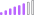cancel
Showing results for
Did you mean:Post Patron

## Calculate distinctcount for ID within quarter based on last month range in quarter

Hi All!

I'm struggling with measure for distinct values of IDs within quarter based on last active month range.

I need to show only 1 value for quater per ID in chart based on range of last active month, tried something like below but I figured out that there some IDs which have only 1st or 2nd month of quarter active and measure below didn't calculate them.

``Calculate( DISTINCTCOUNT(ID), FILTER(DATA, DATA(DATE) = ENDOFQUARTER(DATE) && RANGEID <> BLANK))``

What I need - distinct value per ID per quarter based on last active month range even if range shanged within quarter, please help with changes in measure.
sample of data below:

 ID Period Range 111 1/1/2022 2 111 2/1/2022 1 222 1/1/2022 3 222 2/1/2022 2 222 3/1/2022 3 222 4/1/2022 2

For ID 111 should be feb as last month for q1 with range 1, for 222 should be mar as last month for q1 with range 3 and apr as last month for q2 with range 2.

1 ACCEPTED SOLUTIONCommunity Support

HI @bsas,

Which logic that you used to pick records from same id group? The maximum range or date period?

If you mean the first one, you can use the id to find out the max range first, then use id and range as condition to filter records to get the count.

``````formula =
VAR currDate =
MAX ( Table[Period] )
VAR _range =
CALCULATE (
MAX ( Table[Range] ),
FILTER (
ALLSELECTED ( Table ),
YEAR ( [Period] ) = YEAR ( currDate )
&& QUARTER ( [Period] ) = QUARTER ( currDate )
),
VALUES ( Table[ID] )
)
RETURN
CALCULATE (
DISTINCTCOUNT ( Table[ID] ),
FILTER ( ALLSELECTED ( Table ), [Range] = _range ),
VALUES ( Table[ID] )
)``````

If you mean the second one, you only need to change above formula to get the period, then use it as condition in formula to calculate.

Regards,

Xiaoxin Sheng

Community Support Team _ Xiaoxin
If this post helps, please consider accept as solution to help other members find it more quickly.
4 REPLIES 4Super User

Hi,

Show the expected result very clearly in a Table format.

Regards,
Ashish Mathur
http://www.ashishmathur.comSuper User

@bsas , Have date table with qtr and join with your table and have qtr in visual or filter from date table

calculate(lastnonblankvalue(Table[period]), distinctCOUNT(Table[ID]))

Calendar = Addcolumns(calendar(date(2020,01,01), date(2021,12,31) ), "Month no" , month([date])
, "Year", year([date])
, "Month Year", format([date],"mmm-yyyy")
, "Month year sort", year([date])*100 + month([date])
, "Qtr Year", format([date],"yyyy-\QQ")
, "Qtr", quarter([date])
, "Month",FORMAT([Date],"mmmm")
, "Month sort", month([DAte])
, "Is Today" ,if([Date]=TODAY(),"Today",[Date]&"")
,"Day of Year" , datediff(date(year([DAte]),1,1), [Date], day)+1
, "Month Type", Switch( True(),
eomonth([Date],0) = eomonth(Today(),-1),"Last Month" ,
eomonth([Date],0)= eomonth(Today(),0),"This Month" ,
Format([Date],"MMM-YYYY") )
,"Year Type" , Switch( True(),
year([Date])= year(Today()),"This Year" ,
year([Date])= year(Today())-1,"Last Year" ,
Format([Date],"YYYY")
)
)

!! Microsoft Fabric !!
Microsoft Power BI Learning Resources, 2023 !!
Learn Power BI - Full Course with Dec-2022, with Window, Index, Offset, 100+ Topics !!
Did I answer your question? Mark my post as a solution! Appreciate your Kudos !! Proud to be a Super User! !!Post Patron

Hi @amitchandak
Thanks for your help. I have date table with diff attributes, tried your measure but it is not working as expected, in case ID have 2 diff ranges within quarter it shows this ID twice and I need only last value for quarter e.g. for ID 222 in 1st qarter it shows count 2 with two different rages 2 and 3, should only show count 1 and range 3

created two measures:

``````count1 =  CALCULATE( DISTINCTCOUNT(ID), FILTER( Period, Period[Date] = LASTDATE(Data[Date])))
count2 =  CALCULATE( DISTINCTCOUNT(ID), FILTER( Data, Data[Date] = ENDOFQUARTER(Period[Date])))``````but both give not what I want - 2 2 1 1, maybe this can help?Community Support

HI @bsas,

Which logic that you used to pick records from same id group? The maximum range or date period?

If you mean the first one, you can use the id to find out the max range first, then use id and range as condition to filter records to get the count.

``````formula =
VAR currDate =
MAX ( Table[Period] )
VAR _range =
CALCULATE (
MAX ( Table[Range] ),
FILTER (
ALLSELECTED ( Table ),
YEAR ( [Period] ) = YEAR ( currDate )
&& QUARTER ( [Period] ) = QUARTER ( currDate )
),
VALUES ( Table[ID] )
)
RETURN
CALCULATE (
DISTINCTCOUNT ( Table[ID] ),
FILTER ( ALLSELECTED ( Table ), [Range] = _range ),
VALUES ( Table[ID] )
)``````

If you mean the second one, you only need to change above formula to get the period, then use it as condition in formula to calculate.

Regards,

Xiaoxin Sheng

Community Support Team _ Xiaoxin
If this post helps, please consider accept as solution to help other members find it more quickly.